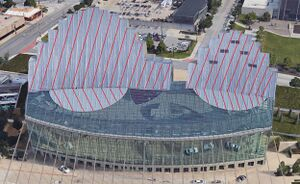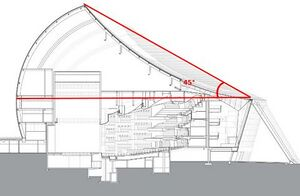This page describes all calculation and assumptions in calculating Kauffman art center retrofit the top roof

## Method

Kauffman art center top viewFig 1: The Muriel Kauffman Center for performing arts, Kansas City. Red hachured area indicates Sought face of the roof. (Google Earth)

To measure hatched area in Fig. 1 Google Earth distance measurement has been used.

• All measurements repeated three times and averaged to minimize the error.
• Google Earth distance measurement tool does all measurement as level plane. This measurement is not accurate for inclined planes like roof.
• to minimize the error angle of the roof was measured and considered in calculations (Fig. 2).
• Top right and left red hatched area in Fig. 1 considered as triangles
• Bottom right and left red hatched area in Fig.1 each estimated to a half circle

### Area calculation of top right and left triangles red hatched in Fig. 1

1. top left:
• base= 63 m
• leveled length (Google Earth)= 31 m
• inclined length (considering 45 degree angled) = 43.84 m
• Top left area= (63 m)*(43.84 m)/2 = 1381 m^2
1. top right
• base= 45.2 m
• leveled length (Google Earth)= 27 m
• inclined length (45 degrees) = 38.18 m
• Top right area= (45.2 m)*(38.18 m)/2= 863 m^2

### Area calculation of bottom right and left half circles red hatched in Fig. 1

1. bottom left
• diameter=30 m
• half circle area= (pi*15^2)/2=350 m^2
1. bottom right
• diameter=39 m
• half circle area= (pi*19.5^2)/2=597 m^2

### Total red hatched area in Fig.1

Total area= 1381 + 863 + 350 + 597 = 3191 (m^2)

Kauffman art center side viewFig. 2 Cross section of Muriel Kauffman Theater (www.inhabit.com))

# Discussion

Cookies help us deliver our services. By using our services, you agree to our use of cookies.